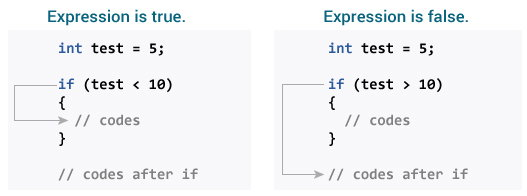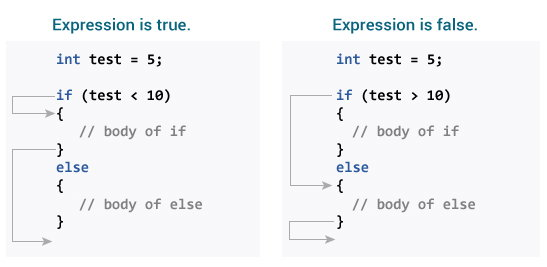# Java if, if...else Statement

In this article, you will learn to use two selection statements: if and if...else to control the flow of your program’s execution.

In programming, it's often desirable to execute a certain section of code based upon whether the specified condition is `true` or `false` (which is known only during the run time). For such cases, control flow statements are used.

## Java if (if-then) Statement

The syntax of if-then statement in Java is:

```if (expression) {
// statements
}
```

Here expression is a boolean expression (returns either `true` or `false`).

If the expression is evaluated to `true`, statement(s) inside the body of `if` (statements inside parenthesis) are executed.

If the expression is evaluated to `false`, statement(s) inside the body of `if` are skipped from execution.

### How if statement works?### Example 1: Java if Statement

``````class IfStatement {
public static void main(String[] args) {

int number = 10;

if (number > 0) {
System.out.println("Number is positive.");
}
System.out.println("This statement is always executed.");
}
}``````

When you run the program, the output will be:

```Number is positive.
This statement is always executed.```

When number is 10, the test expression `number > 0` is evaluated to `true`. Hence, codes inside the body of `if` statements are executed.

Now, change the value of number to a negative integer. Let's say -5. The output in this case will be:

`This statement is always executed.`

When number is -5, the test expression `number > 0` is evaluated to `false`. Hence, Java compiler skips the execution of body of `if` statement.

## Java if...else (if-then-else) Statement

The if statement executes a certain section of code if the test expression is evaluated to true. The `if` statement may have an optional `else` block. Statements inside the body of `else` statement are executed if the test expression is evaluated to `false`.

The syntax of if-then-else statement is:

```if (expression) {
// codes
}
else {
// some other code
}
```

### How if...else statement works?### Example 2: Java if else Statement

``````class IfElse {
public static void main(String[] args) {
int number = 10;

if (number > 0) {
System.out.println("Number is positive.");
}
else {
System.out.println("Number is not positive.");
}

System.out.println("This statement is always executed.");
}
}``````

When you run the program, the output will be:

```Number is positive.
This statement is always executed.```

When number is 10, the test expression `number > 0` is evaluated to `true`. In this case, codes inside the body of `if` are executed, and codes inside the body of `else` statements are skipped from execution.

Now, change the value of `number` to a negative number. Let's say -5. The output in this case will be:

```Number is not positive.
This statement is always executed.```

When number is -5, the test expression `number > 0` is evaluated to `false`. In this case, codes inside the body of `else` are executed, and codes inside the body of `if` statements are skipped from execution.

## Java if..else..if Statement

In Java, it's possible to execute one block of code among many. For that, you can use if..else...if ladder.

```if (expression1)
{
// codes
}
else if(expression2)
{
// codes
}
else if (expression3)
{
// codes
}
.
.
else
{
// codes
}```

The `if` statements are executed from the top towards the bottom. As soon as the test expression is `true`, codes inside the body of that `if` statement is executed. Then, the control of program jumps outside `if-else-if` ladder.

If all test expressions are `false`, codes inside the body of `else` is executed.

### Example 3: Java if..else..if Statement

``````class Ladder {
public static void main(String[] args) {

int number = 0;

if (number > 0) {
System.out.println("Number is positive.");
}
else if (number < 0) {
System.out.println("Number is negative.");
}
else {
System.out.println("Number is 0.");
}
}
}``````

When you run the program, the output will be:

`Number is 0.`

When number is 0, both test expression `number > 0` and `number < 0` is evaluated to `false`. Hence, the statement inside the body of `else` is executed.

The above program checks whether number is positive, negative or 0.

## Java Nested if..else Statement

It's possible to have `if..else` statements inside a `if..else` statement in Java. It's called nested `if...else` statement.

Here's a program to find largest of 3 numbers:

### Example 4: Nested if...else Statement

``````class Number {
public static void main(String[] args) {

Double n1 = -1.0, n2 = 4.5, n3 = -5.3, largestNumber;

if (n1 >= n2) {
if (n1 >= n3) {
largestNumber = n1;
} else {
largestNumber = n3;
}
} else {
if (n2 >= n3) {
largestNumber = n2;
} else {
largestNumber = n3;
}
}

System.out.println("Largest number is " + largestNumber);
}
}``````

When you run the program, the output will be:

`Largest number is 4.5`

Note: In above programs, we have assigned value of variables ourselves to make this easier. However, in real world applications, these values may come from user input data, log files, form submission etc.

You should also check ternary operator in Java, which is kind of shorthand notation of `if...else` statement.